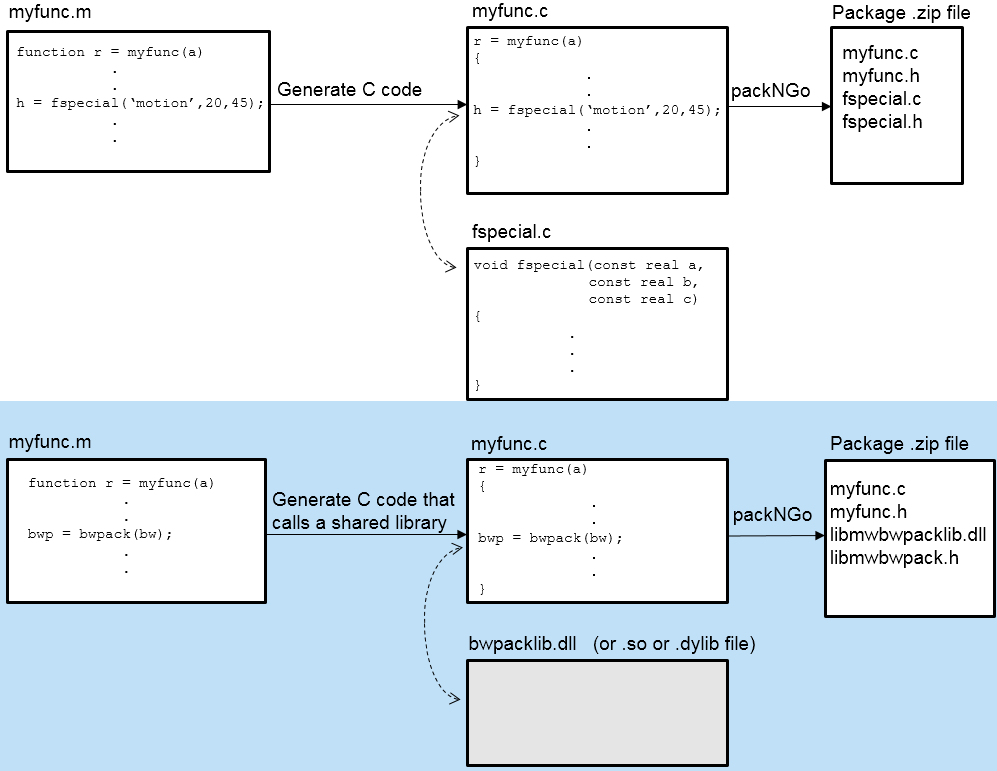## 图像处理的代码生成

### Image Processing Toolbox 中代码生成支持的类型

Image Processing Toolbox 提供三种类型的代码生成支持。

• 生成独立 C 代码的函数。您可以将这些代码合并到在许多平台（例如 ARM 处理器）上运行的应用程序中。仅支持独立 C 代码的一个函数示例是 `immse`

• 生成依赖特定于平台的共享库（`.dll``.so``.dylib`）的 C 代码的函数。使用共享库可保留这些函数中的性能优化，但这会将运行此代码的目标平台限制为只有那些能够托管 MATLAB 的平台。要查看主机平台列表，请参阅系统要求。仅支持依赖共享库的 C 代码的一个函数示例是 `bwpack`

• 根据您在 MATLAB Coder 配置设置中指定的目标平台，生成独立 C 代码或依赖共享库的 C 代码的函数。如果指定通用 `MATLAB Host Computer` 目标平台，则这些函数会生成依赖共享库的 C 代码。如果您指定任何其他目标平台，则这些函数会生成独立的 C 代码。同时支持独立 C 代码和依赖共享库的 C 代码的一个函数示例是 `regionprops`### 使用图像处理函数生成代码

• 使用 Image Processing Toolbox 中的函数，像平常一样编写您的 MATLAB 函数或应用程序。

• 在函数签名的末尾添加 `%#codegen` 编译器指令。此指令指示 MATLAB 代码分析器诊断会阻止成功生成代码的问题。

• 打开 MATLAB Coder (MATLAB Coder)，创建一个工程，并将您的文件添加到工程中。在该 App 中，您可以检查代码的就绪情况以进行代码生成。例如，您的代码可能包含不支持代码生成的函数。针对代码生成进行必需的所有修改。

• MATLAB Coder 的 Generate Code 页上点击 Generate，以生成代码。您可以选择生成 MEX 文件、共享库、动态库或可执行文件。

即使您解决了 MATLAB Coder 标识的所有就绪问题，您仍可能会遇到编译问题。就绪检查只查看函数依存关系。当您尝试生成代码时，MATLAB Coder 可能会发现代码生成不支持的编码模式。请查看错误报告并修改您的 MATLAB 代码，直到编译成功。

## 另请参阅

(MATLAB Coder) | (MATLAB Coder)